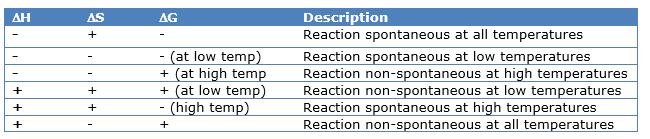# Entropy And Gibbs Functions

## Spontaneity

A spontaneous process which can take place by itself, speed of spontaneous reaction varies from very slow to fast.
Example
Dissolution of Na Cl in water.
CO gets oxidized to CO2 on its own.
A process which can neither take place by itself or by initiation is called non Spontaneous.
Driving forces for spontaneous process: (i) Tendency for minimum energy state. (ii) Tendency for maximum randomness

## Entropy (s)

• It is the measure of degree of randomness or disorderliness of a system.
• Entropy is minimum in solid state and maximum in gaseous state.
• ΔS =Sfinal  - Sinitial
• $\Delta S=\frac {\Delta H}{T}$ Where H is enthalpy and T is temperature
• Entropy in the states of matter is like
Gas>Liquid>Solid
• Entropy Unit is J/K moles

## Second Law of thermodynamics

The Entropy of universe is continuously increasing due to spontaneous processes taking place in it.
ΔSsystem  +ΔSSurrounding  > 0
Stotal > 0
If Stotal  < 0,process is not spontaneous
A spontaneous process cannot be reversed

## Gibb’s Free Energy (ΔG)

It is equal to the minimum amount of useful work done by the system.
Gibbs Functions  (G) is defined as the thermodynamic quantity of the system, the decrease in whose value during a process is equal to the useful work done by the system. It is a extensive property
G= H-TS
The change in Gibbs energy is given by
Mathematically: -
ΔG=ΔH -TΔS
Where G is the Gibbs function
ΔH is the enthalpy change of a reaction,
TΔS is the energy which is not available to useful work. So ΔG is the net energy available to do useful work and is thus a measure of the ‘free energy’
For a process ifΔ G = -ve, reaction is spontaneous
Δ G= 0, reaction is at eqb
Δ G     = +ve, reaction is non – spontaneous
Effects of Temperature on Δ G### Gibbs Energy change in a Reaction

Δr G0r G0 (p) - Δr G0 (r)

### Relation between G and Equilibrium Constant

G = -2.303 RT log Kc
Where Kc = equilibrium constant

### Entropy change  in  a reaction

Δr S0r S0 (p) - Δr S0 (r)

## Third law of Thermodynamics

The Entropy of a perfectly crystalline substance is Zero at zero Kelvin.